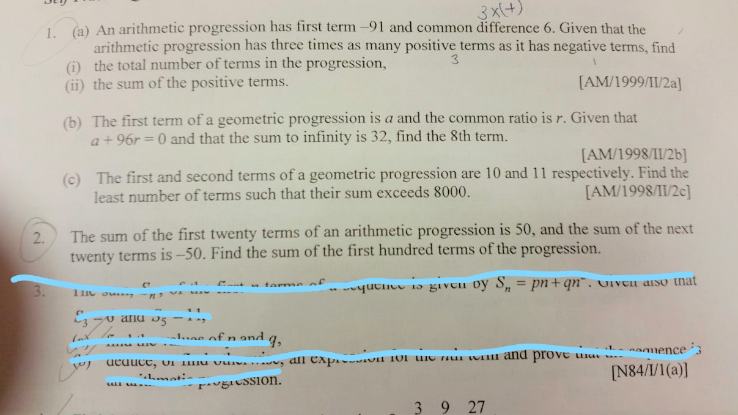# QuestionHI Sir,

Is there possible help to answer 2 questions as attached file.  Please don’t provide solution only. I need more explains how to you figure out.

Thank you.

2) S20 = 50

S40 = 0

solve for a and d.

with that, you can find S100 easily.

0 Replies 0 Likes

c) a = 10, r =11/10

solve Sn>8000

10(1.1^n-1) /(1.1-1) >8000

1.1^n -1>80

1.1^n>81

n lg 1.1 >lg81

n> 46.10

n =47

0 Replies 0 Likes

b) sum to infinity=a/1-r

32= a/1-r

32-32r = a——(1)

given a+96r =0—-(2)

solve for a and r.

r=-1/2 and a =48

sub to find T8.

0 Replies 0 Likes

a=-91; d=6

Tn = -91+(n-1)6

Positive terms: Tn>0

-91+(n-1)6>0

solving the inequality, we get

n>16 1/6

thus T1 to T16 are the negative terms

Given the number of positive terms = 3 times the negative terms

Number of positive terms = 3×16 =48

Total number of terms =48 + 16 =64

Sum of positive terms = T17 +T18 + …. T64 = S64 – S16.

Using the formula to find the sum of n terms in AP, u shld be able to get the solution.

Hope i got it correct.

0 Replies 0 Likes